### Introduction to Index Number - Revision Notes

CBSE Class 11 Economics

Revision Notes
Chapter - 8
INTRODUCTION TO INDEX NUMBER

Introduction to index number: An index number is a statistical device for measuring changes in the magnitude of a group of related variables.

Features of Index Number

1. Index numbers are expressed in terms of percentages. However, percentage sign (%) is never used.
2. Index numbers are relative measurement of group of data.
3. Index numbers offer a precise measurement of the quantitative change in the concerned variables over time.
4. Index number show changes in terms of averages.
5. They are expressed in numbers.
6. Index number facilitates the comparative study over different time period.

Importance of Index number

1. It serves as a barometer for measuring the value of money.
2. Gives knowledge about change in standard of living.
3. It helps the business community in planning their decision.

Limitation of Index Number

1. Limited applicability
2. International comparison is not possible
3. Limited coverage
4. Difficulty in the construction of index number

Types of Index numbers:
(i) Wholesale price index (WPI)
(ii) Consumer price index (CPI) or Cost of living index
(iii) Index of industrial production (IIP)
(iv) Index of Agricultural production (IAP)
(v) Sensex

Methods of constructing index numbers:
1. Construction of Simple Index numbers
a. Simple Aggregative Method
b. Simple Average of Price Relatives Method
2. Construction of weighted Index numbers
a. weighted Average of Price Relative Method
b. Weighted Aggregative Method
Simple aggregative method: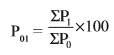Here,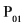= Price index of the current year.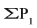= Sum of the prices of the commodities in the current year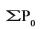= Sum of the prices of the commodities in the base year

Current year: Current year is the year for which average change is to be measured or index of index number is to be calculated.
Base year: Base year is the year of reference from which we want measure extent of change in the current year. The index number of base year is generally assumed to be 100.

Simple average of price Relatives method: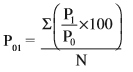Here,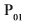= Price index of the current year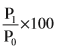= Price relatives
N = Number of commodities

Weighted average of price relative method: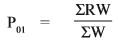Here,= Index number for the current year in relation to base year
W = Weight, R = Price relatives i.e.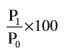Weighted Aggregative method:
(i) Laspeyre’s method  :- P01 = $\frac{\sum {p}_{1}{q}_{0}}{\sum {p}_{0}{q}_{0}}×100$
(ii) Pasche’s method :- P01 = $\frac{\sum {p}_{1}{q}_{1}}{\sum {p}_{0}{q}_{1}}×100$
(iii) Fisher’s Method :- P01 = $\sqrt{\frac{\sum {p}_{1}{q}_{0}}{\sum {p}_{0}{q}_{0}}×\frac{\sum {p}_{1}{q}_{1}}{\sum {p}_{0}{q}_{1}}}×100$

Some Important index numbers:
(i) Consumer price index (CPI)
: CPI is also known as the cost of living index, measures the average change in retail prices.
Methods of constructing CPI:
Here,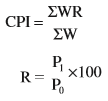W = Weights

(B) Aggregative expenditure method: P01 = $\frac{\sum {p}_{1}{q}_{0}}{\sum {p}_{0}{q}_{0}}×100$
(ii) Wholesale price index (WPI): WPI Indicates the change in the general price level.
(iii) Index of industrial production (IIP): IIP is used to measure the relative increase or decrease in the level of industrial production.
IIP= $\frac{\sum \left[\frac{{q}_{1}}{{q}_{0}}×100\right]W}{\sum W}$
Here,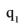= Level of Production in the current year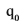= Level of production in the base year
W = Weight
(iv) Index of agriculture production (IAP)
IAP is used to study the rise and fall of the yield of principle crops from one period to other period.
(v) Sensex: Sensex is the short form of Bombay stock exchange sensitive index with 1978-79 as base. It is the benchmark index for the Indian stock market.
It consists or 30 stocks which represent 13 sectors of the economy and the companies are the leaders in their respective industries.

Problems in construction of index numbers:
(i) Purpose of index number.
(ii) Selection of base year.
(iii) Selection of items.
(iv) Selection of the prices of items.
(v) Selection of method of weighting
(vi) Selection of sources of data
(vii) Choice of an average.
(viii) Choice of method.

Uses of index numbers:
(i) To measure the purchasing power of money.
(ii) Knowledge of change in standard of living.
(iii) Adjustment in salaries and allowances.
(iv) Help in framing suitable policies.
(v) As economic barometers.

Inflation and index numbers:
Inflation is described a situation characterised by a sustained increase in the general price level. Generally, inflation is measured in terms of wholesale price index.
Rate of inflation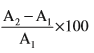Here,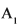= WPI for week first (1)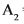= WPI for week second (2)